# area of a regular polygon

Area of a Regular Polygon

The area of a regular polygon is given by the formula below.

area = (½)(apothem)(perimeter)Several other area formulas are also available.

 Regular Polygon Formulasn = number of sidess = length of a sider = apothem (radius of inscribed circle)   R = radius of circumcircle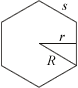Sum of interior angles = (n – 2)·180°Interior angle =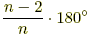Area = (½)nsr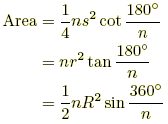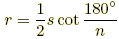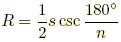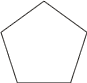Regular Pentagon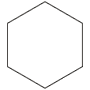Regular Hexagon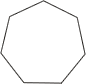Regular Heptagon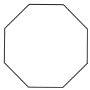Regular Octagon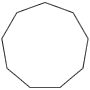Regular Nonagon

See also

Area of a convex polygon

Copyrights © 2013 & All Rights Reserved by nbccc.ushomeaboutcontactprivacy and policycookie policytermsRSS